Try out our new practice tests completely free!# Quiz 15: Active Filters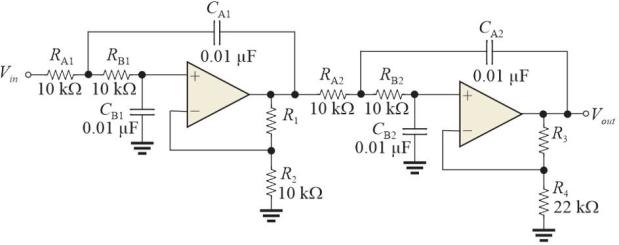Figure 1 Stage 1: DF = 1.848 and R1/R2 = 0.152; stage 2: DF = 0.765 and R3/R4 =1.235. -Refer to Figure 1. The type of filter is a
(Multiple Choice)Figure 1 Stage 1: DF = 1.848 and R1/R2 = 0.152; stage 2: DF = 0.765 and R3/R4 =1.235. -Refer to Figure 1. The ideal roll- off rate is
(Multiple Choice)The damping factor, DF, is determined by the number of poles in a filter.
(True False)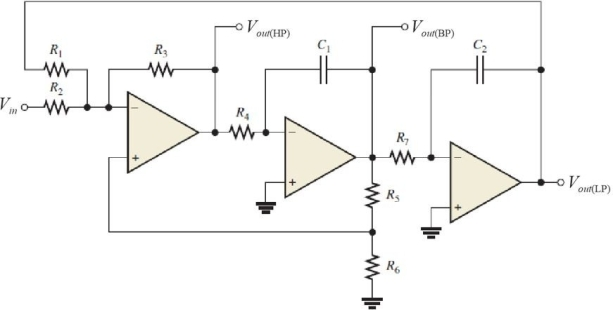Figure 3 -Refer to Figure 3. The last stage is
(Multiple Choice)A basic RC (one R, oneC)filter has a -20dB/decade roll- off.
(True False)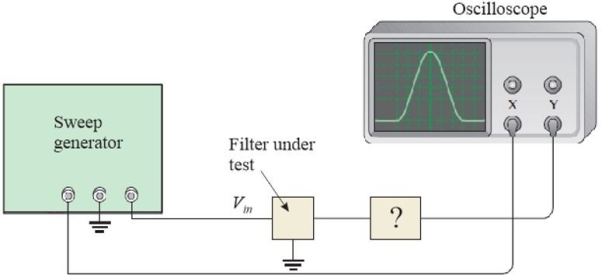Figure 4 A test of a filter using an oscilloscope -Refer to Figure 4. The oscilloscope needs to
(Multiple Choice)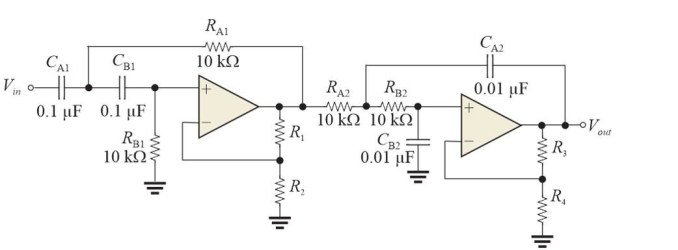Figure 2 Note that the capacitors have different values. -Refer to Figure 2. The circuit is a
(Multiple Choice)A single- pole filter cannot be used as a notch filter.
(True False)The Q of a band- pass filter is inversely proportional to the damping factor.
(True False)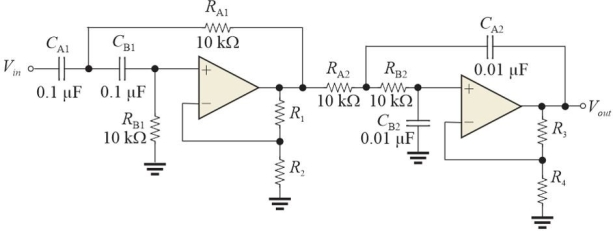Figure 2 Note that the capacitors have different values. -Refer to Figure 2. Assume section 1 and section 2 are exchanged. This will
(Multiple Choice)The Chebyshev filter is noted for its flat response in the passband.
(True False)Figure 2 Note that the capacitors have different values. -Refer to Figure 2. The fc for the second section is
(Multiple Choice)A biquad filter has both low- pass and high- pass outputs.
(True False)The damping factor, DF, affects an active filter's response characteristics.
(True False)The bandwidth of a basic band- pass filter is measured between the center frequency and a critical frequency.
(True False)## Filters

• Essay(0)
• Multiple Choice(0)